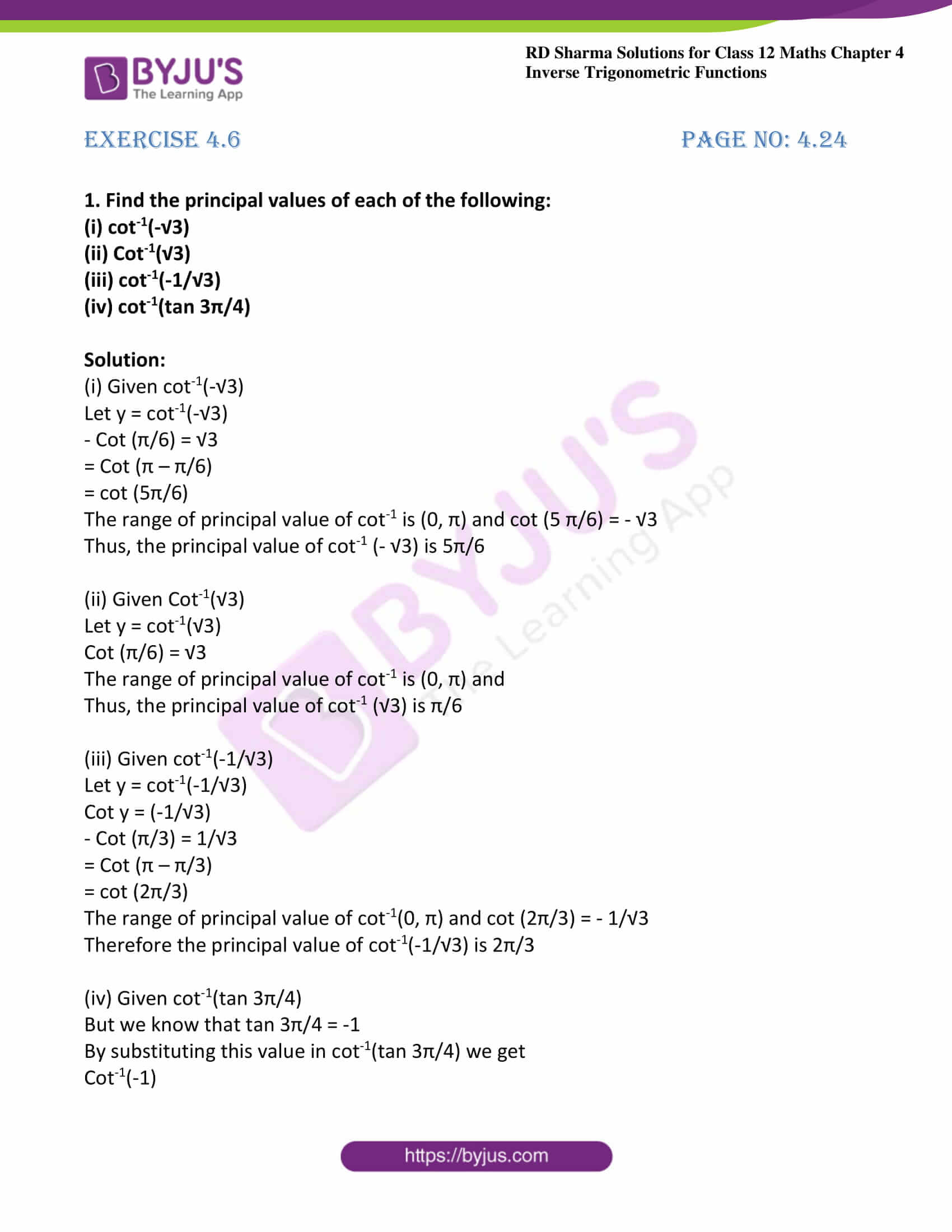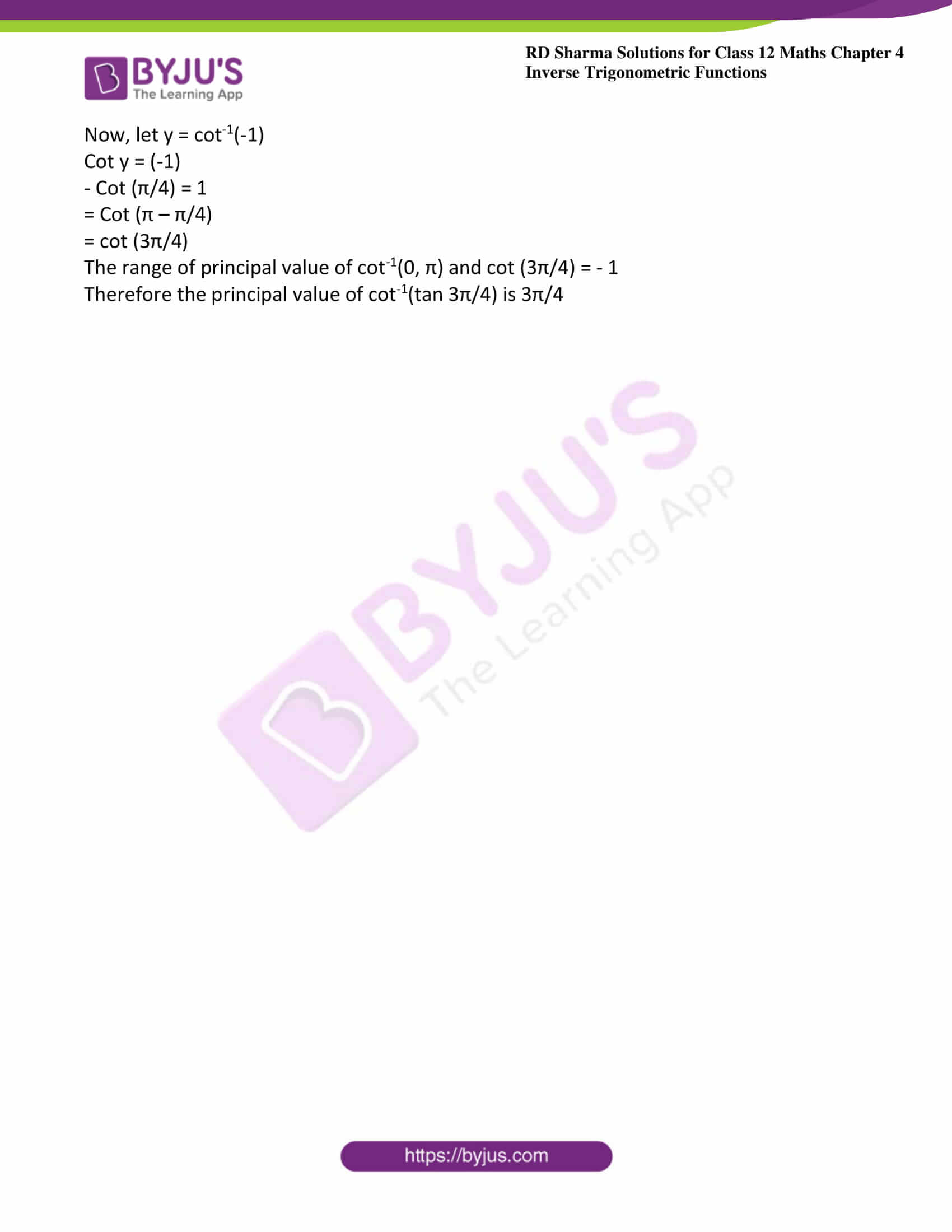# RD Sharma Solutions For Class 12 Maths Exercise 4.6 Chapter 4 Inverse Trigonometric Functions

RD Sharma Solutions Class 12 Maths Exercise 4.6 Chapter 4 Inverse Trigonometric Functions is provided here. Students can make use of solutions PDF while solving exercise wise problems understanding the other possible ways of obtaining answers easily. The solutions are designed by subject matter experts based on the grasping abilities of students. It improves logical thinking skills among students, which are important from the exam point of view.

This exercise is completely based on the inverse of cotangent function. Students who aim to clear the exam with good marks can use RD Sharma Solutions Class 12 Maths Chapter 4 Inverse Trigonometric Functions Exercise 4.6 PDF as a reference guide to boost their exam preparation.

## Download the PDF of RD Sharma Solutions For Class 12 Chapter 4 – Inverse Trigonometric Functions Exercise 4.6### Exercise 4.6 Page No: 4.24

1. Find the principal values of each of the following:

(i) cot-1(-√3)

(ii) Cot-1(√3)

(iii) cot-1(-1/√3)

(iv) cot-1(tan 3π/4)

Solution:

(i) Given cot-1(-√3)

Let y = cot-1(-√3)

– Cot (π/6) = √3

= Cot (π – π/6)

= cot (5π/6)

The range of principal value of cot-1 is (0, π) and cot (5 π/6) = – √3

Thus, the principal value of cot-1 (- √3) is 5π/6

(ii) Given Cot-1(√3)

Let y = cot-1(√3)

Cot (π/6) = √3

The range of principal value of cot-1 is (0, π) and

Thus, the principal value of cot-1 (√3) is π/6

(iii) Given cot-1(-1/√3)

Let y = cot-1(-1/√3)

Cot y = (-1/√3)

– Cot (π/3) = 1/√3

= Cot (π – π/3)

= cot (2π/3)

The range of principal value of cot-1(0, π) and cot (2π/3) = – 1/√3

Therefore the principal value of cot-1(-1/√3) is 2π/3

(iv) Given cot-1(tan 3π/4)

But we know that tan 3π/4 = -1

By substituting this value in cot-1(tan 3π/4) we get

Cot-1(-1)

Now, let y = cot-1(-1)

Cot y = (-1)

– Cot (π/4) = 1

= Cot (π – π/4)

= cot (3π/4)

The range of principal value of cot-1(0, π) and cot (3π/4) = – 1

Therefore the principal value of cot-1(tan 3π/4) is 3π/4

### Access other exercises of RD Sharma Solutions For Class 12 Chapter 4 – Inverse Trigonometric Functions

Exercise 4.1 Solutions

Exercise 4.2 Solutions

Exercise 4.3 Solutions

Exercise 4.4 Solutions

Exercise 4.5 Solutions

Exercise 4.7 Solutions

Exercise 4.8 Solutions

Exercise 4.9 Solutions

Exercise 4.10 Solutions

Exercise 4.11 Solutions

Exercise 4.12 Solutions

Exercise 4.13 Solutions

Exercise 4.14 Solutions